VLOOKUP vs Index Match: What Excel Formula is Better?

People who have used VLOOKUP in Excel for a long while know just how powerful this formula is. You can easily match details of the source information from a huge database and make it easy to fill your spreadsheet and continue on to making pivots for your analysis.

But pundits will argue that Index Match is better. So, what gives? Is VLOOKUP better than Index Match or is it the other way around?

In this VLOOKUP vs Index Match article, we will explore their differences and help you decide which functionality is best for your business needs.

## VLOOKUP vs Index Match: Usefulness and Versatility

Index Match allows the user to look for values based on a database. You can use this formula to look up for values on either rows or columns.

VLOOKUP in Excel, on the other hand, it can only be used to lookup for values on vertically arranged information on a database. Sad to say, but Excel formulas also have limitations.

VLOOKUP will only work on a static column reference. Look at figure 1 below.

Figure 1: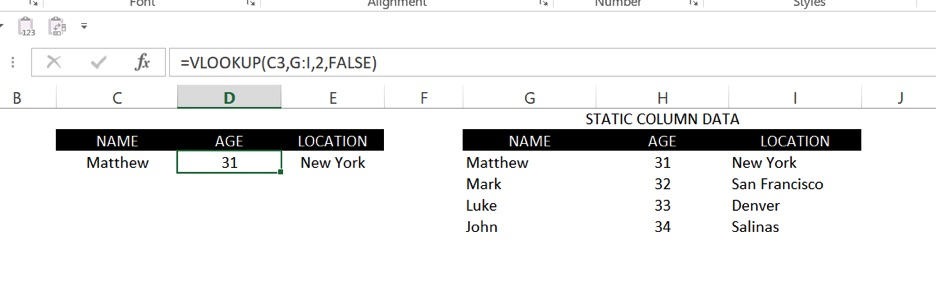In this example, VLOOKUP will only work if your source value in column C matches one in column G. And it will only work if column G is the first or leftmost column in your formula in Microsoft Excel.

An index match, however, uses a dynamic reference. See figure 2 below.

Figure 2:In this example, you can see that you need to get a match from a specific column you selected. In VLOOKUP in Excel, you use a number to tell the program which column the match will come from. This number will lead to errors because it takes only one movement of a column and your entire sheet will go to ruins.

Versatility: Index Match wins one point.

## VLOOKUP vs Index Match: Ease of Use

It is hard to teach an old dog a new trick. As far as the two are compared, the VLOOKUP formula is less complicated than the Index Match. You only have four kinds of syntax in VLOOKUP, which you can lock and drag and adjust per column.

In Index Match, you need to recreate the formula per column and match your data source with the specific column for your return values. This is tedious, to say the least. It also has six components in the formula compared to five in VLOOKUP.

Ease of Use: VLOOKUP wins one point.

## VLOOKUP vs Index Match: Functionality

Now, it is time to find out if VLOOKUP is better than Index Match in terms of functionality. Earlier, we only measured the ease of use as far as understanding the syntax is concerned. Now, if you finally drag and drop the formula, which one will make more sense?

Look at this VLOOKUP formula part A.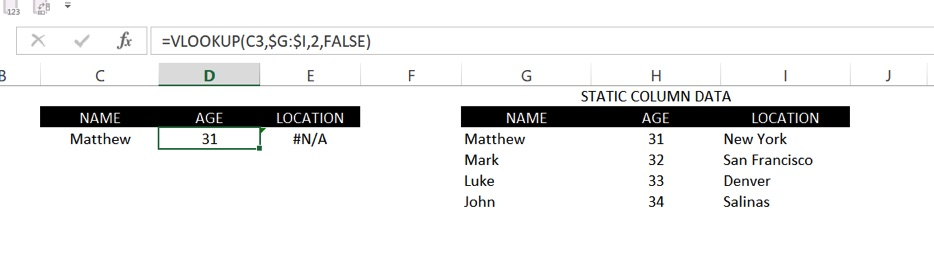And look at Part B.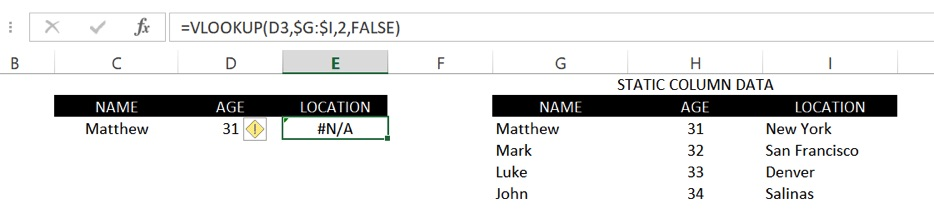In Part A, the formula for the source array is locked. If you copy and paste that formula under Location, it will return a value of an error. The reason for this is that C3 now became D3. VLOOKUP does not know that you are still looking for the value of C3 in reference to your static column data. If you lock it, the problem is you cannot drag it down, or it will just keep returning values for C3. The only solution is to manually change the lookup value, and the column index number on every cell every single time, and then you can drag it down.

Now let’s look at this picture for index Part A.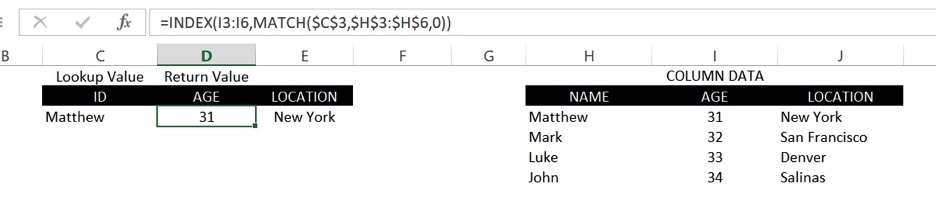And this is Part B.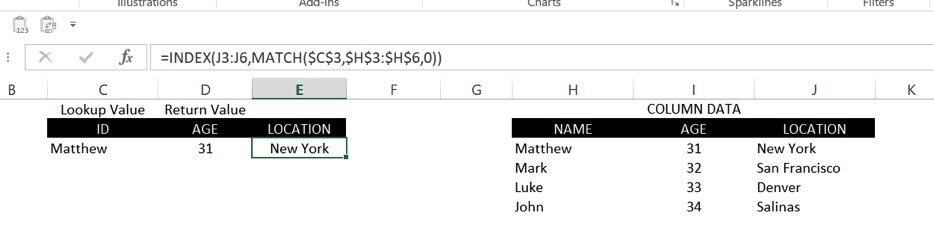If you will observe, we locked C3, which is the lookup value, then we locked H3 to H6, which is the array start, but we did not lock I3 to I6, which is the source of the return value; and since we did not do this, we can copy and paste formula and it will show the desired values without having to toggle it one by one/

Functionality: Index Match wins one point.

## Summary

Clearly, VLOOKUP does not win this battle, if you would only consider the functionality and the versatility. However, VLOOKUP is easier to learn, so it will really depend on the user which one is best for his use.